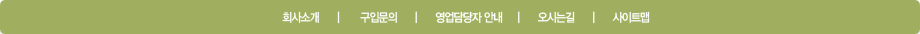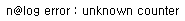통합검색도서명저자명ISBN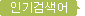Zumdahl,줌달,맥머리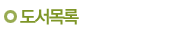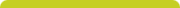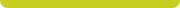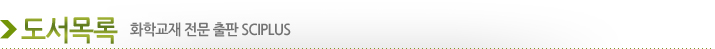현재위치 : 홈 > 도서목록 > 새로나온 도서

 Statistical Methods for the Social Sciences, Global Edition

 지은이 :  Agresti 발행일 :  2017 년 ISBN :  9781292220314 정행가 :  0 원 페이지 :  616 페이지 판행수 :  Global Edition 출판사 :  Pearson

관련도서 보기로스의 확률모형 11판Bayesian Statistics and Marketing

도서소개

For courses in Statistical Methods for the Social Sciences.

Statistical methods applied to social sciences, made accessible to all through an emphasis on concepts Statistical Methods for the Social Sciences introduces statistical methods to students majoring in social science disciplines. With an emphasis on concepts and applications, this book assumes no previous knowledge of statistics and only a minimal mathematical background. It contains sufficient material for a two-semester course. The 5th Edition uses examples and exercises with a variety of “real data.” It includes more illustrations of statistical software for computations and takes advantage of the outstanding applets to explain key concepts, such as sampling distributions and conducting basic data analyses. It continues to downplay mathematics—often a stumbling block for students—while avoiding reliance on an overly simplistic recipe-based approach to statistics.

## Features

• Introduce social science students to statistical methods

o Strong focus on real examples help students learn the fundamental concepts of sampling distributions, confidence intervals, and significance tests.

o Low technical level in first nine chapters makes the text accessible to undergraduate students.

o Integration of descriptive and inferential statistics features from an early point in the text.

o Strong emphasis on regression topics outlines special cases of a generalized linear model, supported by a wide variety of regression models (such as linear regression, ANOVA, logistic, and regression).

o Emphasis on concepts on advanced topics (such as regression and ANOVA) underline the importance of interpreting output from computer packages rather than complex computing formulas.

o NEW! Greater integration of statistical software. Software output shown now uses R and Stata instead of only SAS and SPSS, although much output has a generic appearance. The text appendix provides instructions about basic use of these software packages.

o NEW! Companion website (found at www.pearsonglobaleditions.com/Agresti) now features the data sets analyzed in the text in generic form to copy for input into statistical software. Special directories there also have data files in Stata format and in SPSS format so they are ready for immediate use with those packages. Answers to Select Odd-Numbered Exercises are available at the companion website.

o NEW! New examples and exercises ask students to use applets to help learn the fundamental concepts of sampling distributions, confidence intervals, and significance tests. The text also now relies more on applets for finding tail probabilities from distributions such as the normal, t, and chi-squared. The excellent applets cited, can be found at www.pearsonglobaleditions.com/Agresti.

•   Emphasis on concepts and applications
•   Descriptive statistic chapter gives students early exposure to contingency tables, regression, concepts of association, and response and explanatory variables.
•   Relative frequency concept is introduced in chapter four and briefly summarizes three basic probability rules occasionally applied in the text.
•   Full focus on t distribution makes results consistent with software output and emphasizes that the normality assumption for the t distribution is mainly needed for small samples with one-sided inference.
•   Confidence intervals present methods for proportion before the mean. This allows students to learn the basic concept of a confidence interval without being confronted with too many topics all at once.
•   Comparing Two Groups chapter introduces ideas of bivariate analysis, highlights the distinction between response and explanatory variables, defines independent and dependent samples, discusses how to compare two groups with a difference or a ratio of two parameters, and shows the general formula for finding a standard error of a difference between two independent estimates.
•   ANOVA focus explains the ideas behind the F test and gives examples before presenting the sums of square formulas.
•   UPDATED! Regression modeling chapter now has a new section using case studies to illustrate how research studies commonly use regression with both types of explanatory variables. The chapter also has a new section introducing linear mixed models.
•   Logistic regression is explained in a less technical way so it’s widely understood by all students.

## New to this Edition

·    Greater integration of statistical software. Software output shown now uses R and Stata instead of only SAS and SPSS, although much output has a generic appearance. The text appendix provides instructions about basic use of these software packages.

·    New examples and exercises ask students to use applets to help learn the fundamental concepts of sampling distributions, confidence intervals, and significance tests. The text also now relies more on applets for finding tail probabilities from distributions such as the normal, t, and chi-squared. The excellent applets cited, can be found at www.pearsonglobaleditions.com/Agresti.

·    ANOVA coverage has been reorganized to put more emphasis on using regression models with dummy variables to handle categorical explanatory variables.

·    Companion website (found at www.pearsonglobaleditions.com/Agresti) now features the data sets analyzed in the text in generic form to copy for input into statistical software. Special directories there also have data files in Stata format and in SPSS format so they are ready for immediate use with those packages. Answers to Select Odd-Numbered Exercises are available at the companion website.

·    Chapter 5 has a new section that introduces maximum likelihood estimation and the bootstrap method.

·    Chapter 13 on regression modeling now has a new section using case studies to illustrate how research studies commonly use regression with both types of explanatory variables. The chapter also has a new section introducing linear mixed models.

·    Chapter 14 contains a new section on robust regression covering standard errors and nonparametric regression.

도서차례
 <저자 및 역자 소개> body{font-family: Tahoma,Verdana,Arial;font-size: 11px;color: #555555;margin: 0px}td{font-size :11px; font-family: Tahoma,Verdana,Arial;}p{margin-top:1px;margin-bottom:1px;} body{font-family: Tahoma,Verdana,Arial;font-size: 11px;color: #555555;margin: 0px}td{font-size :11px; font-family: Tahoma,Verdana,Arial;}p{margin-top:1px;margin-bottom:1px;}
 <목차> body{font-family: Tahoma,Verdana,Arial;font-size: 11px;color: #555555;margin: 0px}td{font-size :11px; font-family: Tahoma,Verdana,Arial;}p{margin-top:1px;margin-bottom:1px;} body{font-family: Tahoma,Verdana,Arial;font-size: 11px;color: #555555;margin: 0px}td{font-size :11px; font-family: Tahoma,Verdana,Arial;}p{margin-top:1px;margin-bottom:1px;} PrefaceAcknowledgmentsIntroduction Sampling and Measurement Descriptive Statistics Probability Distributions Statistical Inference: Estimation Statistical Inference: Significance Tests Comparison of Two Groups Analyzing Association between Categorical Variables Linear Regression and Correlation Introduction to Multivariate Relationships Multiple Regression and Correlation Regression with Categorical Predictors: Analysis of Variance Methods Multiple Regression with Quantitative and Categorical Predictors Model Building with Multiple Regression Logistical Regression: Modeling Categorical ResponsesAppendix: R, Stata, SPSS, and SAS for Statistical AnalysesAnswers to Select Odd-Numbered ExercisesBibliographyCreditsIndex